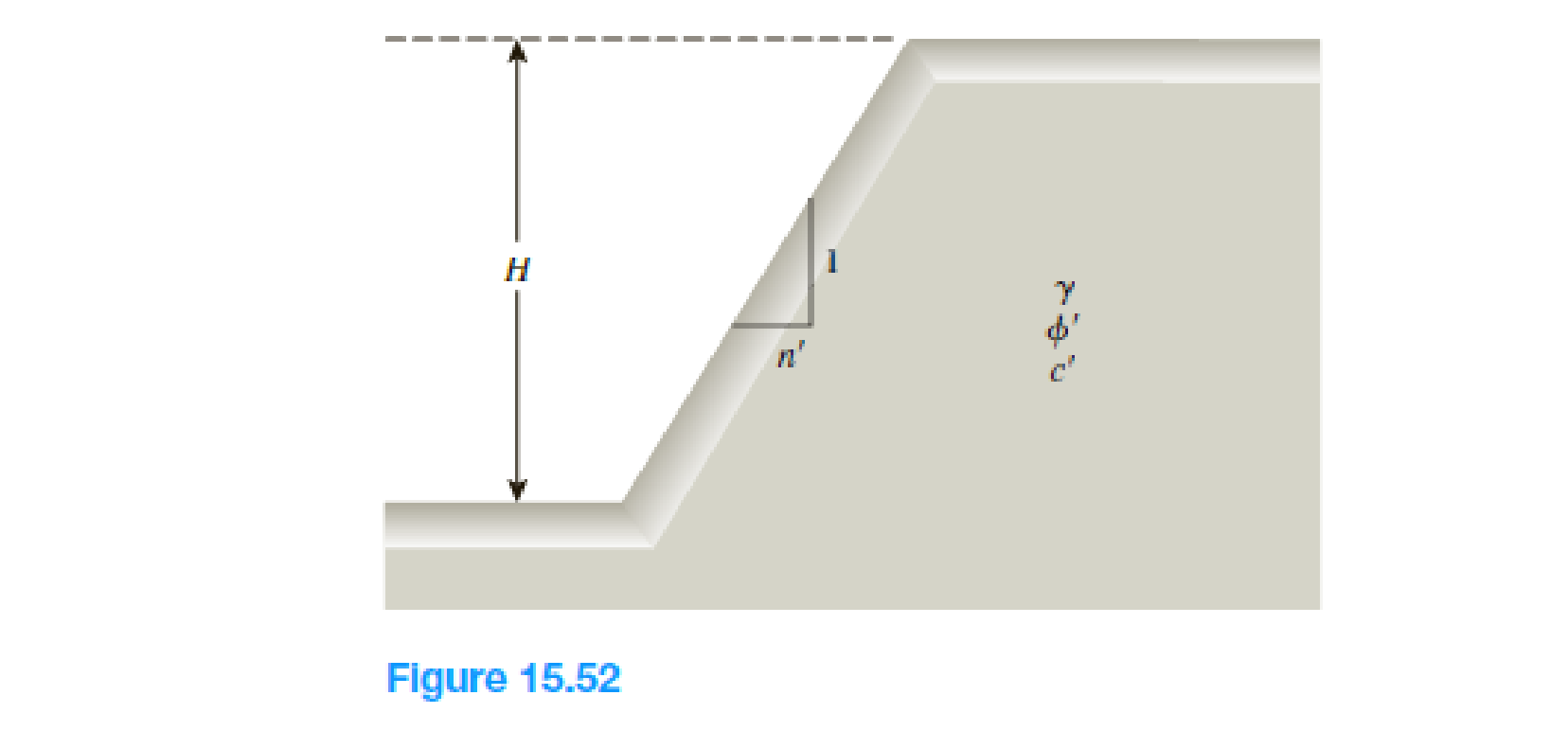Chapter 15, Problem 15.21PPrinciples of Geotechnical Enginee...

9th Edition
Braja M. Das + 1 other
ISBN: 9781305970939

Solutions

Chapter
SectionPrinciples of Geotechnical Enginee...

9th Edition
Braja M. Das + 1 other
ISBN: 9781305970939
Textbook Problem

Refer to Figure 15.52. Using Michalowski’s solution given in Figure 15.25 (ϕ' > 0), determine the critical height of the slope for the following conditions. a. n' = 1.5, ϕ' = 15°, c' = 1075 lb/ft2, and γ = 121 lb/ft3 b. n' = !, ϕ' = 21°, c' = 43 kN/m2, and γ = 18 kN/m3(a)

To determine

Find the critical height Hcr of the slope using Michalowski’s solution.

Explanation

Given information:

The stability coefficient n is 1.5.

The angle of friction ϕ is 15°.

The cohesion c is 1,075lb/ft2.

The unit weight γ of the soil is 121lb/ft3.

Calculation:

Determine the inclination angle β using the formula.

β=tan1(1n)

Substitute 1.5 for n.

β=tan1(11.5)=33.69°

Determine the value of Fstanϕ.

Take the value of factor of safety as 1.0 for critical height.

Substitute 1.0 for Fs and 15° for ϕ.

Fstanϕ=1.0tan15°=3.73

Refer Figure (15.25) “Michalowski’s analysis for stability of simple slopes” in the text book

(b)

To determine

Find the critical height Hcr of the slope using Michalowski’s solution.

Still sussing out bartleby?

Check out a sample textbook solution.

See a sample solution

The Solution to Your Study Problems

Bartleby provides explanations to thousands of textbook problems written by our experts, many with advanced degrees!

Get Started

What does the term 3 Vs refer to?

Database Systems: Design, Implementation, & Management

Label the parts of the surface grinder.

Precision Machining Technology (MindTap Course List)

What should be done with a leaking cylinder if the leak cannot be stopped?

Welding: Principles and Applications (MindTap Course List)